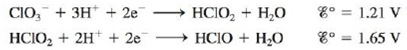# A disproportionation reaction involves a substance that acts as both an oxidizing and a reducing agent, producing higher and lower oxidation states of the same element in the products. Which of the following disproportionation reactions are spontaneous under standard conditions? Calculate Δ G° and K at 25°C for those reactions that are spontaneous under standard conditions. a. 2 Cu + ( a q ) → Cu 2 + ( a q ) + Cu ( s ) b. 3 Fe 2 + ( a q ) → 2 Fe 3 + ( a q ) + Fe ( s ) c. HClO 2 ( a q ) → ClO 3 − ( a q ) + HClO ( a q ) (unbalanced) Use the half-reactions:### Chemistry: An Atoms First Approach

2nd Edition
Steven S. Zumdahl + 1 other
Publisher: Cengage Learning
ISBN: 9781305079243

#### Solutions

Chapter
Section### Chemistry: An Atoms First Approach

2nd Edition
Steven S. Zumdahl + 1 other
Publisher: Cengage Learning
ISBN: 9781305079243
Chapter 17, Problem 86E
Textbook Problem
1 views

## A disproportionation reaction involves a substance that acts as both an oxidizing and a reducing agent, producing higher and lower oxidation states of the same element in the products. Which of the following disproportionation reactions are spontaneous under standard conditions? Calculate ΔG° and K at 25°C for those reactions that are spontaneous under standard conditions.a. 2 Cu + ( a q ) → Cu 2 + ( a q ) + Cu ( s ) b. 3 Fe 2 + ( a q ) → 2 Fe 3 + ( a q ) + Fe ( s ) c. HClO 2 ( a q ) → ClO 3 − ( a q ) + HClO ( a q ) (unbalanced)Use the half-reactions:(a)

Interpretation Introduction

Interpretation:

The information regarding the disproportionation reaction is given. Various questions based on the spontaneity are to be answered and the calculation of ΔG° and K is to be stated.

Concept introduction:

The relationship between reduction potential and standard reduction potential value and activities of species present in an electrochemical cell at a given temperature is given by the Nernst equation.

The value of Ecell is calculated using Nernst formula,

E=E°(RTnF)ln(Q)

At room temperature the above equation is specified as,

E=E°(0.0591n)log(Q)

To determine: The spontaneity of the given reaction (a) and further calculation of ΔG° and K .

### Explanation of Solution

Given

The disproportionation reaction is,

2Cu+(aq)Cu2+(aq)+Cu(s)

The reaction taking place at cathode is,

Cu+(aq)+eCu(s)E°red=0.52V

The reaction taking place at anode is,

Cu+(aq)Cu2+(aq)+eE°ox=0.16V

Add both the oxidation and reduction half-reaction,

Cu+(aq)+eCu(s)Cu+(aq)Cu2+(aq)+e

The final equation is,

2Cu+(aq)Cu2+(aq)+Cu(s)

The overall cell potential is calculated as,

E°cell=E°ox+E°red=0.16V+0.52V=0.36V

The relationship between cell potential and Gibbs free energy change is given by the formula,

ΔG°=nFE°cell

Where,

• ΔG° is the Gibbs free energy change at the standard conditions.
• n is the number of electrons involved in the reaction.
• F is the Faraday’s constant.
• E°cell is the cell potential at the standard condition.

As the value of E°cell comes out to be positive then ΔG° becomes negative. Therefore it leads to a spontaneous reaction.

The relationship between cell potential and Gibbs free energy change is given by the formula,

ΔG°=nFE°cell

The reaction involves the transfer of 1mole

Substitute the values of n , E°cell and F in the above equation as,

ΔG°=1×96,485×0

(b)

Interpretation Introduction

Interpretation:

The information regarding the disproportionation reaction is given. Various questions based on the spontaneity are to be answered and the calculation of ΔG° and K is to be stated.

Concept introduction:

The relationship between reduction potential and standard reduction potential value and activities of species present in an electrochemical cell at a given temperature is given by the Nernst equation.

The value of Ecell is calculated using Nernst formula,

E=E°(RTnF)ln(Q)

At room temperature the above equation is specified as,

E=E°(0.0591n)log(Q)

To determine: The spontaneity of the given reaction (b) and the value of ΔG° and K if the reaction is spontaneous.

(c)

Interpretation Introduction

Interpretation:

The information regarding the disproportionation reaction is given. Various questions based on the spontaneity are to be answered and the calculation of ΔG° and K is to be stated.

Concept introduction:

The relationship between reduction potential and standard reduction potential value and activities of species present in an electrochemical cell at a given temperature is given by the Nernst equation.

The value of Ecell is calculated using Nernst formula,

E=E°(RTnF)ln(Q)

At room temperature the above equation is specified as,

E=E°(0.0591n)log(Q)

To determine: The spontaneity of the given reaction (c) and further calculation of ΔG° and K if the reaction is spontaneous.

### Still sussing out bartleby?

Check out a sample textbook solution.

See a sample solution

#### The Solution to Your Study Problems

Bartleby provides explanations to thousands of textbook problems written by our experts, many with advanced degrees!

Get Started

Find more solutions based on key concepts
Little fat digestion takes place in the stomach. T F

Nutrition: Concepts and Controversies - Standalone book (MindTap Course List)

Which of the following is an alkaline earth metal? a. Na b. Mg c. Sc d. Ti

Chemistry for Today: General, Organic, and Biochemistry

A young woman buys an inexpensive used car stock car racing. It can attain highway speed with an acceleration o...

Physics for Scientists and Engineers, Technology Update (No access codes included)

How does the latitude of a coastal city affect the tides thereor does it?

Oceanography: An Invitation To Marine Science, Loose-leaf Versin

Why are features like the Moons maria not observed on Mercury?

Foundations of Astronomy (MindTap Course List)